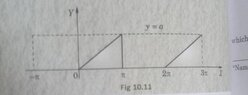# {Edit-Solved} Confirmation requested on deriving functions from graphs

• I
• warhammer
In summary, the graph shows a periodic function with a period of 2##\pi##. The function can be expressed as ##y=\frac{a}{\pi}(x-2n\pi)## for ##0<(x-2n\pi)<\pi## for integer n and ##y=\frac{a}{\pi}x## for ##2n\pi<x<(2n+1)\pi## for integer n. To find the Fourier Series for this function, one can use the formula ##f(x)=\frac{a_0}{2}+\sum_{k=1}^\infty (b_k \sin kx + a_k \cos kx)## and calculate

#### warhammer

TL;DR Summary
So I basically saw this graph specified as a particular waveform in my book while reading Fourier Series. I decided to try and derive its function since once I do that I can easily find the FS. Please find the photo and my attempt below, just need a small confirmation if I'm right/wrong.
So I thought that the graph tries to tell us that the function is periodic after 2π interval. So I tried to derive its function from the graph as follows using the point slope equation form for the points (0,0) & (a,π): ##y= ({a}/{π})*x##

I hope this function is alright and I just need to find its Fourier Series

#### Attachments

•IMG_20220313_022657_845.jpg
21.2 KB · Views: 52
Actually the graph shows
##y=\frac{a}{\pi}(x-2n\pi)## for ##0<(x-2n\pi)<\pi## for integer n
y=0 for others.

•warhammer
anuttarasammyak said:
Actually the graph shows
##y=\frac{a}{\pi}x## for ##2n\pi<x<(2n+1)\pi## for integer n
y=0 for others.
Oh I see. Now I realize how you have represented it an even general manner. Although I am a bit confused now, how should I find FS for the same, I mean I'm confused about the intervalbin which integration for FS will be carried out..

Because the function, say f(x), has period of 2##\pi##, it is expressed as
$$f(x)=\frac{a_0}{2}+\sum_{k=1}^\infty (b_k \sin kx + a_k \cos kx)$$
You may calculate ##a_k## and ##b_k## in a usual way.

•warhammer
anuttarasammyak said:
Because the function, say f(x), has period of 2##\pi##, it is expressed as
$$f(x)=\frac{a_0}{2}+\sum_{k=1}^\infty (b_k \sin kx + a_k \cos kx)$$
You may calculate ##a_k## and ##b_k## in a usual way.
Thank you so much! Now I get it•anuttarasammyak and berkeman